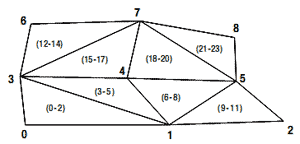# 5.3 使用索引移除冗余顶点

## 问题## 解决方案## 工作原理

private void InitVertices()
{
vertices = new VertexPositionColor;
vertices= new VertexPositionColor(new Vector3(0, 0, 0), Color.Red);
vertices= new VertexPositionColor(new Vector3(1, 0, 0), Color.Green);
vertices= new VertexPositionColor(new Vector3(2, 0, 1), Color.Blue);
vertices= new VertexPositionColor(new Vector3(0, 1, -1), Color.Orange);
vertices= new VertexPositionColor(new Vector3(1, 1, 0), Color.Olive);
vertices= new VertexPositionColor(new Vector3(2, 1, 0), Color.Magenta);
vertices = new VertexPositionColor(new Vector3(0, 2, 0), Color.Yellow);
vertices = new VertexPositionColor(new Vector3(1, 2, 1), Color.Tomato);
vertices = new VertexPositionColor(new Vector3(2, 2, -1), Color.Plum);
myVertexDeclaration = new VertexDeclaration(device, VertexPositionColor.VertexElements);
}

private int[] indices;

private void InitIndices()
{
indices = new int;

indices = 0;
indices = 3;
indices = 1;

indices = 1;
indices = 3;
indices = 4;

indices = 1;
indices = 4;
indices = 5;

indices = 1;
indices = 5;
indices = 2;

indices = 3;
indices = 6;
indices = 7;

indices = 3;
indices = 7;
indices = 4;

indices = 4;
indices = 7;
indices = 5;

indices = 5;
indices = 7;
indices = 8;
}

basicEffect.World = Matrix.CreateScale(2.0f);
basicEffect.View = fpsCam.ViewMatrix;
basicEffect.Projection = fpsCam.ProjectionMatrix;
basicEffect.VertexColorEnabled 	= true;
basicEffect.Begin();
foreach (EffectPass pass in basicEffect.CurrentTechnique.Passes)
{
pass.Begin();
device.VertexDeclaration = myVertexDeclaration;
device.DrawUserIndexedPrimitives<VertexPositionColor>(PrimitiveType.TriangleList, vertices, 0, 9, indices, 0, 8);
pass.End();
}
basicEffect.End();

## 代码

2006 - 2023，推荐分辨率1024*768以上，推荐浏览器Chrome、Edge等现代浏览器，截止2021年12月5日的访问次数：1872万9823 站长邮箱